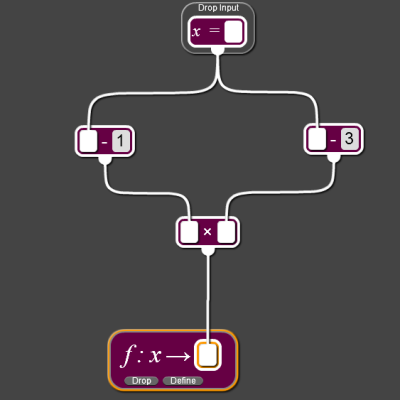#### You may also like### Converse

Clearly if a, b and c are the lengths of the sides of an equilateral triangle then a^2 + b^2 + c^2 = ab + bc + ca. Is the converse true?### Consecutive Squares

The squares of any 8 consecutive numbers can be arranged into two sets of four numbers with the same sum. True of false?### Parabolic Patterns

The illustration shows the graphs of fifteen functions. Two of them have equations y=x^2 and y=-(x-4)^2. Find the equations of all the other graphs.

##### Age 14 to 16Challenge Level

The mapping below was created using the NRICH Number Plumber.

Click here to watch a video explaining how to use the Number Plumber.

Click on the mapping to explore the relationship between the function and its graph.Now replace the 1 and 3 with other values. How does this affect the graph?